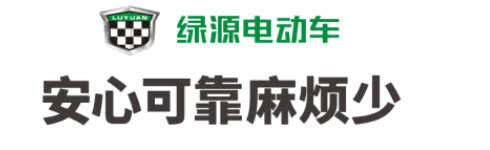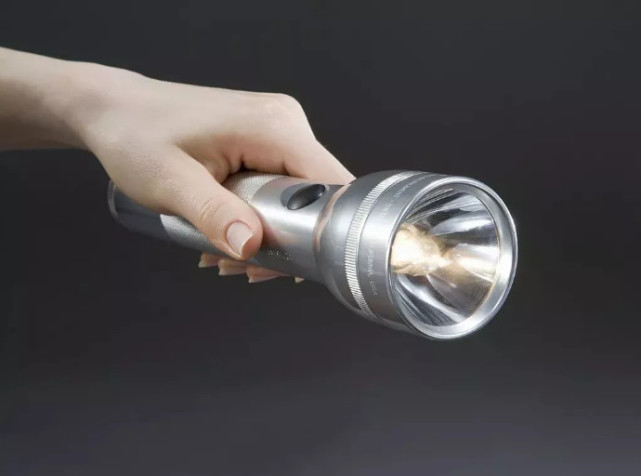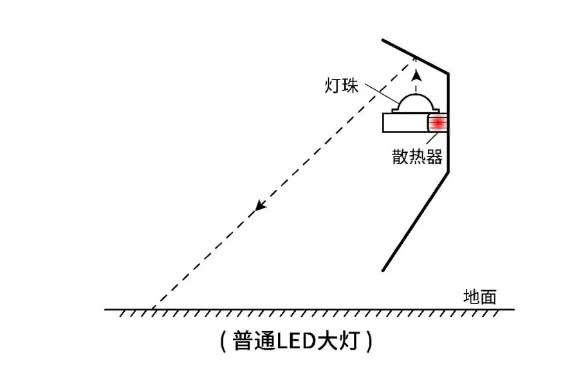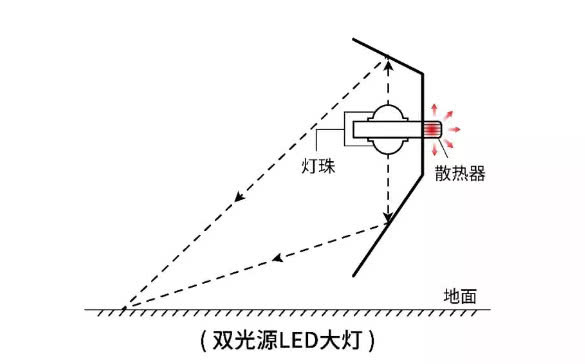## 绿源“最亮”新技术来袭，为夜间骑行安全加分！

2019年01月29日 10:18 来源：车行天下 超过：11688次关注如果用星级来表示重要性,5星代表非常重要,那么关于夜间骑行要开大灯这件事,懂车弟表示:事关生命,可以打6颗星。

然而,现在市场上的灯具质量良莠不齐。一般电动车所用的LED灯,很多都存在亮度不足、光线涣散、明暗截止线模糊等问题。有的灯具散热效果差,天气冷的时候,水汽很容易就会凝结在灯罩上让大灯变模糊。严重的时候,还会导致内部塑件软化变形,甚至引发火灾等电路安全事故。

那么,为什么会存在这些问题?回答这个问题,首先我们需要了解一下大灯的工作原理。你可能没有近距离地观察过电动车的大灯,但你一定用过手电筒当我们打开手电筒的开关,手电筒灯罩内部的发光源开始发光,大部分光线照射到图中银色的反射罩上,经过反射罩的反射汇聚成一束,再照射出去。而电动车大灯的工作原理,便与之类似。发光源可以说是决定亮度的最主要因素,从下面的示意图可以看出,普通大灯只有上面一个发光源,导致了亮度不足,光线涣散。而且散热器位于大灯内部,热量无法及时有效地散发出去,由此有了散热效果差的问题。普通LED大灯结构示意图

既然明白了问题所在,绿源便从源头解决问题,研发出了双光源LED大灯,真正做到了亮度强,光线集中,明暗截止线清晰并且散热效果良好。绿源双光源LED大灯结构示意图

对比两张示意图可以看出,相较于普通大灯,绿源的双光源大灯的下方多了一个发光源。同时,反射罩的角度经过精密的计算和设计,可以确保将上下两个发光源发出的光反射到同一个区域,由此大大增加了光照强度。

为了提高散热效果,双光源大灯将散热器置于灯罩外部,这样就可以将产生的热量及时有效地散发出去,防止其在内部堆积,从而避免了寒冷天气水汽在灯罩表面凝结、内部塑件软化变形等问题。不仅保障了行车安全,也大大延长了大灯的使用寿命。

为了更直观地看到两者的差异,让我们来看一个测试大灯亮度的视频。

视频中,测试绿源双光源LED大灯时,测光仪显示的数据为849,而普通大灯只有601,足以说明绿源大灯的亮度。冬天天亮得晚、黑得早,绿源双光源LED大灯,照亮你上班下班的路。

最后,让我们再通过一个视频,直观地感受一下普通大灯灯珠和绿源双光源LED大灯灯珠的区别,以及大灯在电动车上的实际使用效果。

绿源双光源LED大灯,闪到你了吗?

#### 相关文章

0-500 字已有评论 0条 查看评论>>

### 热门标签

﻿
• 快速找车
• 选择品牌
• 选择品牌
• A  奥迪
• A  阿斯顿·马丁
• A  阿尔法·罗密欧
• B  宝沃
• B  布加迪
• B  巴博斯
• B  保时捷
• B  宾利
• B  奔驰
• B  宝马
• B  本田
• B  别克
• B  标致
• B  比亚迪
• B  宝骏
• B  北汽制造
• B  北汽新能源
• B  北汽幻速
• B  北汽威旺
• B  北京汽车
• B  奔腾
• B  北汽绅宝
• C  长安
• C  长安商用
• C  长城
• C  昌河
• D  大众
• D  道奇
• D  DS
• D  东南
• D  东风风神
• D  东风风行
• D  东风小康
• D  东风风度
• D  东风
• F  福特
• F  丰田
• F  菲亚特
• F  法拉利
• F  福田
• F  福迪
• F  福汽启腾
• G  观致
• G  广汽传祺
• G  广汽吉奥
• G  GMC
• H  红旗
• H  汉腾汽车
• H  哈弗
• H  哈飞
• H  海格
• H  海马
• H  华颂
• H  黄海
• H  华泰
• H  恒天
• J  吉利汽车
• J  捷豹
• J  Jeep
• J  江淮
• J  江铃
• J  金杯
• J  九龙
• J  金旅
• K  凯翼
• K  凯迪拉克
• K  克莱斯勒
• K  科尼塞克
• K  卡威
• K  开瑞
• L  路虎
• L  林肯
• L  劳斯莱斯
• L  兰博基尼
• L  雷克萨斯
• L  铃木
• L  雷诺
• L  理念
• L  力帆
• L  莲花汽车
• L  猎豹
• L  路特斯
• L  陆风
• M  马自达
• M  MG
• M  MINI
• M  玛莎拉蒂
• M  摩根
• M  迈凯轮
• N  纳智捷
• O  欧宝
• O  讴歌
• O  欧朗
• Q  奇瑞
• Q  起亚
• Q  启辰
• R  日产
• R  荣威
• R  瑞麒
• S  三菱
• S  斯威汽车
• S  萨博
• S  smart
• S  斯柯达
• S  斯巴鲁
• S  思铭
• S  双龙
• S  上汽大通
• S  双环
• T  特斯拉
• T  腾势
• W  沃尔沃
• W  五菱汽车
• W  五十铃
• W  威兹曼
• W  威麟
• X  现代
• X  雪佛兰
• X  雪铁龙
• X  西雅特
• Y  一汽
• Y  英菲尼迪
• Y  英致
• Y  依维柯
• Y  野马汽车
• Y  永源
• Z  众泰
• Z  中华
• Z  中兴
• Z  知豆
• 选择车系
• 选择车系
• 车型对比
• 选择品牌
• 选择品牌
• A  奥迪
• A  阿斯顿·马丁
• A  阿尔法·罗密欧
• B  宝沃
• B  布加迪
• B  巴博斯
• B  保时捷
• B  宾利
• B  奔驰
• B  宝马
• B  本田
• B  别克
• B  标致
• B  比亚迪
• B  宝骏
• B  北汽制造
• B  北汽新能源
• B  北汽幻速
• B  北汽威旺
• B  北京汽车
• B  奔腾
• B  北汽绅宝
• C  长安
• C  长安商用
• C  长城
• C  昌河
• D  大众
• D  道奇
• D  DS
• D  东南
• D  东风风神
• D  东风风行
• D  东风小康
• D  东风风度
• D  东风
• F  福特
• F  丰田
• F  菲亚特
• F  法拉利
• F  福田
• F  福迪
• F  福汽启腾
• G  观致
• G  广汽传祺
• G  广汽吉奥
• G  GMC
• H  红旗
• H  汉腾汽车
• H  哈弗
• H  哈飞
• H  海格
• H  海马
• H  华颂
• H  黄海
• H  华泰
• H  恒天
• J  吉利汽车
• J  捷豹
• J  Jeep
• J  江淮
• J  江铃
• J  金杯
• J  九龙
• J  金旅
• K  凯翼
• K  凯迪拉克
• K  克莱斯勒
• K  科尼塞克
• K  卡威
• K  开瑞
• L  路虎
• L  林肯
• L  劳斯莱斯
• L  兰博基尼
• L  雷克萨斯
• L  铃木
• L  雷诺
• L  理念
• L  力帆
• L  莲花汽车
• L  猎豹
• L  路特斯
• L  陆风
• M  马自达
• M  MG
• M  MINI
• M  玛莎拉蒂
• M  摩根
• M  迈凯轮
• N  纳智捷
• O  欧宝
• O  讴歌
• O  欧朗
• Q  奇瑞
• Q  起亚
• Q  启辰
• R  日产
• R  荣威
• R  瑞麒
• S  三菱
• S  斯威汽车
• S  萨博
• S  smart
• S  斯柯达
• S  斯巴鲁
• S  思铭
• S  双龙
• S  上汽大通
• S  双环
• T  特斯拉
• T  腾势
• W  沃尔沃
• W  五菱汽车
• W  五十铃
• W  威兹曼
• W  威麟
• X  现代
• X  雪佛兰
• X  雪铁龙
• X  西雅特
• Y  一汽
• Y  英菲尼迪
• Y  英致
• Y  依维柯
• Y  野马汽车
• Y  永源
• Z  众泰
• Z  中华
• Z  中兴
• Z  知豆
• 选择车系
• 选择车系
• 选择车型
• 选择车型
• 意见反馈